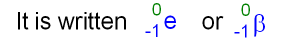gcsescience.com                                       7                                       gcsescience.com

Beta Particles.

What is a Beta Particle?

A beta particle (also written as β-particle) is the same as an electron.
It is often called a high energy electron because it is very fast moving.
A beta particle comes from the nucleus of an atom! It has a
charge of  -1 and very little mass (only 1 ÷ 1840 as big as a proton).The e shows that the particle is an electron.
A beta particle is given a mass number of zero because the
mass is very small compared to a proton or a neutron.

What are the Properties of a Beta
Particle?

1. A beta particle has a negative charge because it is an electron.

2. A beta particle has less mass than an alpha particle but
more mass than a gamma ray. See the three types of radioactivity.
This means that

(i) beta particles have more penetrating ability than alpha particles
but less penetrating ability than
gamma rays.

(ii) beta particles are less ionising than alpha particles but more
ionising
than gamma rays.

Where do
Beta Particles come from?

Beta particles may be emitted from the nucleus during the
decay of either big or small nuclei. See some examples.

How can the Direction of a
Beta Particle be Changed?

The negative charge on the beta particle means that it will be
deflected by a magnetic field or an electric field (like the charged
droplets in an inkjet printer). A beta particle will be deflected
more than an alpha particle (because of its smaller mass)
and in the opposite direction (because of its negative charge).

gcsescience.com     Physics Quiz     Index     Radioactivity Quiz     gcsescience.com